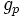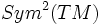# Riemannian metric

This article defines a tensor (viz a section on a tensor bundle over the manifold) of type (0,2)

## Definition

### Given data

A differential manifold$M$.

### Definition part

A Riemannian metric$g$ on$M$ associates to every point$p \in M$ a positive definite symmetric bilinear form$g_p$, such that$g_p$ varies smoothly with$p$.

### Tensoriality

A Riemannian metric is a$(0,2)$-tensor, viz a section of the$(0,2)$-tensor bundle. In fact, it is a section of the bundle$Sym^2(TM)$, viz the bundle of symmetric 2-tensors.

## Flow

Given a differential manifold, there could be many Riemannian metrics on it. A flow of a metric on the differential manifold is a Riemannian metric that gradually evolves with time.